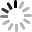18．修一段6千米的路，7天可完成，4天可修（　　）
 A． $\frac{1}{7}$ B． $\frac{3}{2}$ C． $\frac{4}{7}$ D． $\frac{2}{3}$

$\frac{1}{7}$×$4=\frac{4}{7}$．